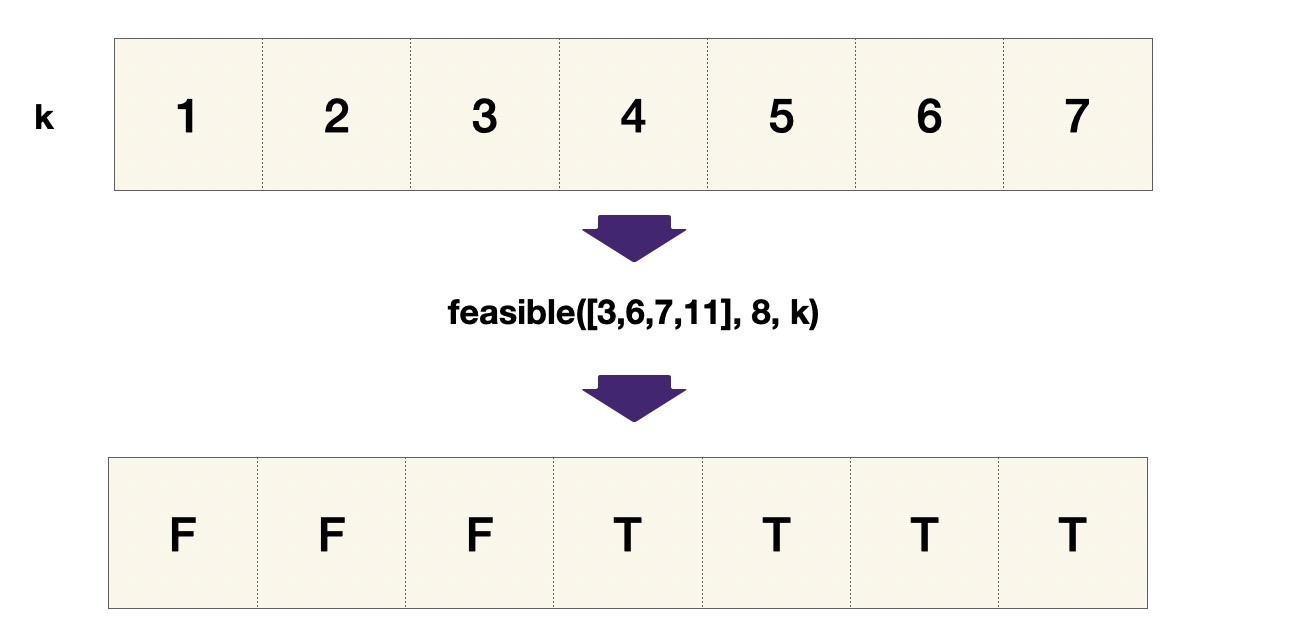# LeetCode Koko Eating Bananas Solution

Koko loves to eat bananas. There are `n` piles of bananas, the `ith` pile has `piles[i]` bananas. The guards have gone and will come back in `h` hours.

Koko can decide her bananas-per-hour eating speed of `k`. Each hour, she chooses some pile of bananas and eats `k` bananas from that pile. If the pile has less than `k` bananas, she eats all of them instead and will not eat any more bananas during this hour.

Koko likes to eat slowly but still wants to finish eating all the bananas before the guards return.

Return the minimum integer `k` such that she can eat all the bananas within `h` hours.

Example 1:
Input: `piles = [3,6,7,11], h = 8`
Output: `4`

Example 2:
Input: `piles = [30,11,23,4,20], h = 5`
Output: `30`

Example 3:
Input: `piles = [30,11,23,4,20], h = 6`
Output: `23`

Constraints:

• `1 <= piles.length <= 104`
• `piles.length <= h <= 109`
• `1 <= piles[i] <= 109`

## Solution

We want to apply the binary search template, and implement the `feasible` function. Here, the feasible function is whether Koko `can_finish_eating` all piles within `h` hours while eating at speed `k` per hour.

Since Koko eats at only one pile during each hour, `ceil(float(p)/k)` is the time Koko takes to finish pile `p`. Note that `p/k` does not work here because we want a whole number of hours so we needed to round up `p/k`. Therefore, the feasiblity is determined by whether Koko's `hours_used` is within `h` hours, where `hours_used` is the total hours to finish all piles.

Recall example 1 with Input: `piles = [3,6,7,11], h = 8`, and Output: `4`, the `can_finish_eating` function returns the following results.#### Implementation

``````1def can_finish_eating(piles, h, k):
2    hours_used = 0
3    for p in piles:
4        hours_used += ceil(float(p)/k)
5    return hours_used <= h
6
7def minEatingSpeed(piles, h):
8    left, right = 1, 1000000000 # 10^9 max length of the piles
9    ans = -1
10    while left <= right:
11      mid = (left + right) // 2
12      if can_finish_eating(piles, h, mid):
13          ans = mid
14          right = mid - 1
15      else:
16          left = mid + 1
17    return ans``````